Reach Us+44-1522-440391
Trying to Explicit Proofs of Some Veys Theorems in Linear Connections | OMICS International
Journal of Generalized Lie Theory and Applications
All submissions of the EM system will be redirected to Online Manuscript Submission System. Authors are requested to submit articles directly to Online Manuscript Submission System of respective journal.

# Trying to Explicit Proofs of Some Veys Theorems in Linear Connections

Lantonirina LS*

Department of Mathematics and Computer Sciences, University of Antananarivo, Madagascar

*Corresponding Author:
Lantonirina LS
Department of Mathematics and Computer Sciences,
University of Antananarivo, Madagascar
Tel: 261334651397
E-mail: [email protected]

Received Date: January 23,2016; Accepted Date: February 15,2016; Published Date: March 15,2016

Citation: Lantonirina LS (2016) Trying to Explicit Proofs of Some Vey’s Theorems in Linear Connections. J Generalized Lie Theory Appl S2:009. doi:10.4172/1736- 4337.S2-009

Copyright: © 2016 Lantonirina LS. This is an open-access article distributed under the terms of the Creative Commons Attribution License, which permits unrestricted use, distribution, and reproduction in any medium, provided the original author and source are credited.

Visit for more related articles at Journal of Generalized Lie Theory and Applications

#### Abstract

Let Χ a diferentiable paracompact manifold. Under the hypothesis of a linear connection r with free torsion Τ on Χ, we are going to give more explicit the proofs done by Vey for constructing a Riemannian structure. We proposed three ways to reach our object. First, we give a sufficient and necessary condition on all of holonomy groups of the connection ∇ to obtain Riemannian structure. Next, in the analytic case of Χ, the existence of a quadratic positive definite form g on the tangent bundle ΤΧ such that it was invariant in the infinitesimal sense by the linear operators ∇k R, where R is the curvature of ∇, shows that the connection ∇ comes from a Riemannian structure. At last, for a simply connected manifold Χ, we give some conditions on the linear envelope of the curvature R to have a Riemannian structure

#### Keywords

Linear connections; Riemannian connection; Levi-civita connection; Holonomy groups; Linear en-velope; Kthderivations; Lie algebras

#### Preliminary and Introduction

In 1978 Vey was the examinator of Anona's phd at Institut Fourier Grenoble. In this time, Vey was written some theorems in linear connections. The title of this unpublished paper was: "Sur les connexions riemanniennes" means "On the Riemannian connections". They had discussions, as said Vey, this result was not well explicit. So, one of the motivations of this paper is to explicit some of them about linear connections. First, we consider a paracompact manifoldΧ, we are looking for a Riemannian metric g which is invariant by parallel transport such that it produces a linear connection∇ with ∇g = 0. Next, we assume that Χ is a simply connected real analytic manifold accompanied by a real analytic connection∇, these new assumptions construct us a positive definite quadratic form g (satisfying ∇g = 0) infinitesimally preserved by the infinitesimal holonomy group. This is obtaining by the fact that the Lie algebra of holonomy groups coincides with the Lie algebra of the infinitesimal holonomy group. Finally, we present our problem so as to consider the linear envelope of the curvature, in the case where X is a smooth manifold. However, under these conditions that the linear envelope of the curvature of constant dimension coincides with the Lie algebra of the holonomy group, make the quadratic form g parallel to∇. Thus builds a Riemannian structure. Recently, several authors treat the same questions as R. Feres in and A. Vanzurava [1,2]. This last author gave an algorithm for constructing Riemannian structure which is similar as Veydone in the end of his paper. This redaction gives an interest for a next one where we use some results and idea of the present paper. So, let us recall some useful definitions in linear connections.

Definition 1

First, let X is a smooth manifold,Τx X, x ∈ X the vector space of tangent vectors on x and Τ X the tangent bundle defined by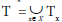Recall that a Riemannian manifold X is a smooth manifold equipped with a Riemannian structure g on X which is defined by the morphism of bundles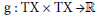such that ∀x∈X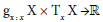defined an inner product with ∀ U,V ∈ ΤΧ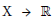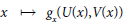is differentiable.

Definition 2

Let X be a smooth manifold, let Γ(X) be the module of vector fields on X and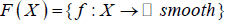the ring of real smooth functions. We recall that a linear connection on X is the application

: Γ(X) × Γ(X) → Γ(X)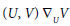such that, ∀ U, U’, V, V’∈ Γ(X), f∈F (X) we have:

i.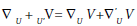ii.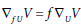iii.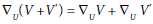iv.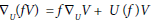Theorem 3

(Fundamental Theorem of Riemannian Geometry) Let X be a Riemannian manifold. Any Riemannian structure g produces an unique linear connection ∇ called Levi-Civita's connection on X with free torsion such that ∇g=0.

#### The Holonomy Groups and Riemannian Structure

Definition 4

Let X be a smooth manifold, a path is a smooth function from [0,1] to X.

Definition 5

let X be a smooth manifold, x a point of X . We call holonomy groups based on a point x of

the Levi-Civita's connection ∇, or Riemannian holonomy, denoted by Η ο l (x) the set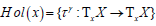where γ : [0, 1] →X is closed path along x to x such that γ(0)= γ(1)=x

Proposition 6

Let X be a smooth manifold, and x, y ∈ X . If X is connected and γ a path x to y, the

holonomy groups of the point x, y are isomorphic and we have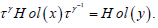Definition 7

Let K be a compact group. We call that an Haar measure on K denoted μ is the unique invariant measure by right and left translation in K, then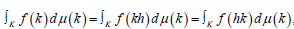such that f∈L(K) a ring of all functions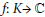.

Definition 8

Let X be a Riemannian manifold, g0 a metric on X, K a compact group. We define an invariant metric g on X which normalizes any metric g0 of X on the compact group K. Let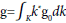Where k* is the “pull-back “of k.

This integration follows the Haar measure property.

Proposition 9

Recall that all paracompact manifolds X admit a Riemannian metric .

Proposition 10

Let X be a smooth manifold and the linear connection on X with free torsion. ∇ proceeds from a Riemannian structure if and only if its holonomy groups are relatively compact.

Proof.

On the one hand, suppose ∇ comes from a Riemannian structure (X, g).Then by the Fundamental Theorem of Riemannian Geometry, we have the Levi-Civita's connection ∇ on X. Consequently, the Riemannian holonomy is well defined by the holonomy group of Levi- Civita's connection on X. But, the holonomy group is a subgroup of the orthogonal group O (n) which is compact, so this subgroup is relatively compact.

On the other hand, let us suppose that the holonomy group on each point of x € X is relatively compact. Let g0 be a Riemannian metric on the paracompact manifold X, and x € X. Then, it leaves invariant a positive definite quadratic form g on a point x of X on TX. Indeed, we have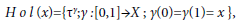then we define,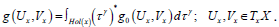Let ,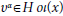then we have,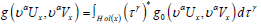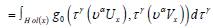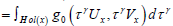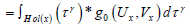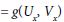Let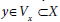(Vx neighbourhood of x). Since X a smooth manifold, then X is locally homeomorphic to an open set of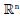. Otherwise,is locally connected, and locally connected is preserved by homeomorphism. Consequently, under the Proposition 6, the holonomy is independent of the selected base point. Then, the parallel transport relatively to ∇ of the quadratic form g along the path from x to y is independent of the path, by definition of holonomy. Thus, it constructs a Riemannian structure g on X preserved by parallel transport, with ∇g = 0. Since ∇ is of free torsion, and uniqueness of ∇, and then ∇ is a Riemannian connection.

#### Real analytic manifold and Riemannian structure

For the following, we suppose X a simply connected manifold to have an explicit result.

Definition 11

Let x a point of X, denoted by C(x) the set of closed curves on x, and C0(x) the set of the contractible curves on point x. Take a point u∈ p-1(x), where p is the projection of the linear structure bundle L(X) at X cf. . We recall that the holonomy group on x denoted by ψ( x) is the subgroup of the diffeomorphism p-1(x) whose the element are obtained by parallel transport of the curves in C(x), that is to say, the element of ψ( x) are of the form τ : p-1(x)→ψ0(x), →τ∈C(x). In the similar ways, we call holonomy group restricted on point x denoted ψ0( x) the subgroup of diffeomorphism of p-1 (x) whose the element are of the form τ : p-1(x), ∀τ∈C0(x).

• For u∈p-1(x), we define holonomy groups on point u byψ0 (u)={a∈Gl(n,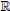),Ra(u)= τ(u), for τ∈ψ(x)}∈Gl(n,).The same, we define the restricted holonomy groups on point u .

Now, let us define the local holonomy group by ψ*(u)=∩ψ0(u, Uk) where ψ0(u, Uk) is a subset of ψ0(u) such that ψ0(u, Uk)⊂ψ0(u, Uk+1), with Uk indicates a sequence the neighbourhood of x satisfied Uk+1∈ Uk, for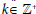and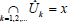Proposition 12

Let X be a smooth manifold, L(X) a linear structure fiber on X, H a connection on L(X) .

Then, all tangent vectors U of TuL(X) is called vertical (resp. horizontal) when it belong to Vu (resp. Hu), u ∈ L(X), where Vu (resp. Hu) indicate the vertical (resp. horizontal) subspace of TuL(X).

Definition 13

Let m0(u) a subspace of g l (n,) generated by Ωu(U; V )(the curvature form on L(X) cf.  p.152) the horizontal vectors U; V ∈ Tu(L(X)) with u ∈ L(X). By recurrence on k, mk(u) defined a subspace of gl(n.) generated by the elements of mk-1 (u) and the element of the form V1 _ _ _ _ _ Vk(Ω(U; V )), where U; V; V1,…, Vk are the horizontal vectors. Let g’ (u) the union of all the mk(u); k = 0,1, 2,… . The set of g’ (u) is a sub-algebra of gl(n;) and the connected Lie subgroup generated by g’ (u) is called infinitesimal holonomy groups on point u of L(X). We denote it by ψ’(u).

For a real analytic manifold, denoted by Η ο l (x) resp. Η ο l (x), resp. Η ο l (x) the holonomy groups (resp. the restricted holonomy group, resp. the infinitesimal holonomy groups) on point x in X.

Proposition 14

Let X be a real analytic manifold, the linear real analytic connection . Denoted by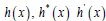the respective Lie algebras of holonomy groups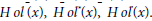The two groups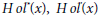constitute a Lie subgroups of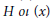namely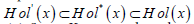and con-sequently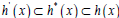Since X is a real analytic manifold, we have the reverse inclusion for the holonomy groups and the equality between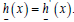Theorem 15

Let X be a real analytic manifold,∇ the linear real analytic connection associated to X .

The Lie algebra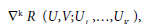where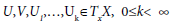and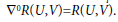Proposition 16

Let (X;∇) be a real analytic simply connected, with free torsion, x a point of X, g a

symmetric bilinear quadratic form on TxX . Then the invariance of g by Η ο l (x) is characterized by: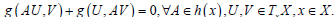(1)

Proposition 17

Let X be a real analytic manifold, simply connected, ∇ an analytic connection with free torsion

on X and R its curvature. Let x be point of X. If there exist a positive definite quadratic form g on TxX, preserved in the infinitesimal sens by all of linear operators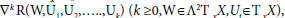then ∇ proceeds from a Riemannian structure.

Proof.

Let x ∈ X, g a positive definite quadratic form on TxX, ∇ the analytic connection associated X , R the curvature of the connection ∇. Suppose that g is preserved in the infinitesimal sense by all linear operators ∇k R(W,U1,U2,…..,Uk)

of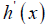(according to the Theorem 15), that is to say that we have according to the

Propositions 16 and 14.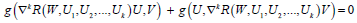(2)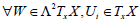and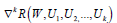(k integer ≥ 0) in h’(x): Since and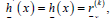then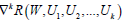belongs to the linear envelope in the endomorphism of TxX. Then the equation (2) comes,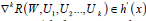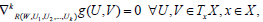therefore ∇kg = 0 for all k ≥ 0, it follows that ∇g = 0. Since the torsion is supposed null, then ∇ comes from a Riemannian structure

#### Linear envelope and Riemannian structure

Lemma 18

Let E be a real vector space of finite dimension, and I an interval of, and Vi (t) a sequence of vectors which depend differentially on t ∈ I. Let L(t) the vector space spanned by Vi(t). Suppose that L(t) of constant dimension, and for all i and for all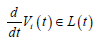then L(t) is independent of t.

Proof.

We proceed like the arguments of proof in  pp. 943-944.

Theorem 19

(Ambrose-Singer Theorem) The Lie algebra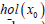of the holonomy groups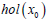(Lie sub-algebra of the Lie algebra g) are spanned by the vectors Ωx(U,V ) for all point x of a principal bundle P cf., link with x0 by a piecewise smooth curve of extremity x and x0 and U, V thehorizontal vectors on x .

Proposition 20

Let X be a smooth manifold, and a linear connection of X. If the tensor ∇R takes all values in the linear envelope of the curvature, and if it has a constant dimension, then it coincides with the Lie algebra of the holonomy groups in each point.

Proof.

Let x a point of X. Let us indicate by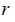the linear envelope of the curvature on x ∈ X; and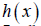the Lie algebra of the holonomy. Let y a point of X, and τ γthe parallel transport along (on End (TX)), where γ indicates a path joins x and y. According to the Ambrose- Singer theorem,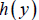is the linear envelope of subspaces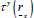Take then a point x ∈ X, a path (t) is parametrized in [0; 1] and joins x to y. Recall that R is defined by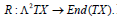Let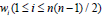a basis of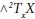and, wi(t) the basis of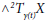obtained by parallel transport along of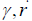defined a linear envelope in End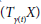of the operators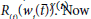by hypothesis ∇ R takes its values on the linear envelope of the curvature, then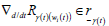Let us consider the subspace of End (TyX)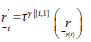' since the linear envelope is supposed of constant dimension, then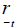has an independent dimension that is to say independent of t, and it defines the linear envelope of the operators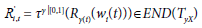It result from precedent that for all t ∈ [0, 1];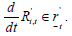(3)

Since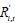depends differentially of t for t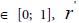has a constant dimension by hypothesis, and that we have the relation (3). Then according to the Lemma18,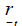t is independent of t. Then,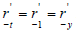Consequently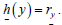Theorem 21 (Lie-Palais) 

Let X be a compact smooth manifold and we have an action on X by the Lie algebra of finite dimension, then this is a lift of an action of Lie group of finite dimension.

Proposition 22

Let X be a simply connected manifold, ∇ a linear connection on X with free torsion, and R its curvature. Supposed that the free conditions which follow are verified:

(a) the linear envelope of the curvature R has a constant dimension,

(b) the tensor R takes its values in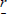(c) in all points x of X, there exists a positive definite quadratic form on TxX infinitesimally preserved by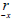,then the connection∇ proceeds from a Riemannian structure on X.

Proof.

Let x a point of X, suppose verified the above hypothesis. Since (a) and (b) are true by hypothesis, then according to the Proposition 20, the linear envelope coincides with the Lie algebra of the holonomy group. Consequently, the linear envelope is stable by the Lie bracket and then admits a Lie algebra structure. Now according to the condition (c), there exist a quadratic positive definite form on TxX preserved infinitesimally byIt follows that the linear envelope is compact. We deduce there that the Lie algebra of holonomy groups is of finite dimension see condition (a). Since X is locally compact, then according to Lie-Palais theorem, the Lie algebra of the group is the lift of the holonomy group whose the topologic structure compact is preserved. Therefore, the holonomy group is relatively compact. Now the Proposition 10 says that for X be a smooth manifold, ∇ its connection with free torsion, ∇ comes from a Riemanniann structure if and only if its holonomy group are relatively compact. Then we have the result.

#### References

Select your language of interest to view the total content in your interested language

### Article Usage

• Total views: 8638
• [From(publication date):
specialissue-2016 - Sep 16, 2019]
• Breakdown by view type
• HTML page views : 8472

## Post your commentCan't read the image? click here to refresh
###### Peer Reviewed Journals

Make the best use of Scientific Research and information from our 700 + peer reviewed, Open Access Journals

International Conferences 2019-20

Meet Inspiring Speakers and Experts at our 3000+ Global Annual Meetings

Top